The equilibrium constant, Kc

Introduction:

• In AS we covered the characteristics of an equilibrium:

Characteristics of the equilibrium state

1)   Equilibrium can only be established in a closed system.  Matter cannot be exchanged with the surroundings (this will affect the position of the equilibrium), but energy can be exchanged.

2)   Equilibrium can be approached from either direction.  The products can be used as the reactants to set up the equilibrium – reversible reactions.

3)   Equilibrium is a dynamic state – At equilibrium the rate in both directions must be the same.

4)   Dynamic equilibrium is stable under fixed conditions but is sensitive to changes in temperature, pH, pressure.

• In AS we discovered that Le Chatelier’s Principle was given as a way of determining how changes in the conditions can affect the extent to which a reaction will go.

• A more Quantitative approach is needed to understand more fully the ideas of equilibria

The equilibrium law:

• The equilibrium law is an expression of the amounts of products : reactants in an equilibrium.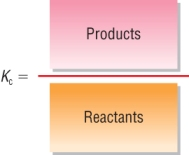Kc = [PRODUCTS]p [REACTANTS]r
• Consider the reaction:

 aA + bB D cC + dD
• Where [A], [B], [C] and [D] are the equilibrium concentrations of reactants and products
• The indices a,b,c and d are the stoichiometric numbers in the balanced chemical reaction
 Kc = [C]c    [D]d [A]a    [B]b
• This expression gives us a mathematical way of looking at the proportions of products and reactants in an equilibrium.
• At equilibrium these relative proportions will not change.
• This means a constant is produced, this is given by - Kc

Approaching equilibrium

• Consider the reversible reaction:
 N2O4(g) D 2NO2(g)
• N2O4 is a colourless gas, NO2 is a brown gas.
 Initially: N2O4 molecules decompose into 2 NO2 molecules,  the rate of the forward reaction is fast.  Because there are very few molecules of NO2, the reverse reaction can only happen slowly. As the reaction proceeds: There are now fewer N2O4 molecules available to decompose, the rate of the forward reaction decreases. There are now more NO2 molecules present so the rate of the reverse reaction increases. At equilibrium: Eventually the forward reaction takes place at exactly the same rate as the reverse reaction.  Dynamic equilibrium is established and is denoted by  D The concentrations of reactants and products now remain constant.
• Graph shows how the concentrations of reactants and products change: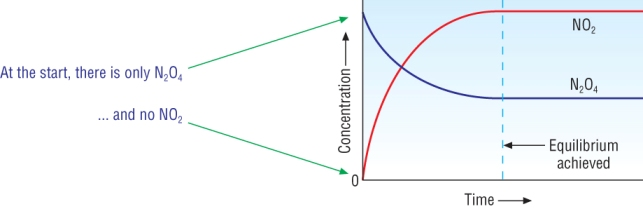Writing expressions for Kc:

• At equilibrium the concentrations of NO2 and N2O4 are constant:
 N2O4(g) D 2NO2(g)
 Kc = [NO2]2 [N2O4]
• A few other examples:
 2SO2(g) + O2(g) D 2SO3(g)
 Kc = [SO3]2 [SO2]2   [O2]

 H2(g) + I2(g) D 2HI(g)
 Kc = [HI]2 [H2]   [I2]

Units of Kc

• These have to be worked out for each Kc
• Just like the rate constant, put the units into each expression:
 Kc = [NO2]2 Kc = [SO3]2 Kc = [HI]2 [N2O4]1 [SO2]2   [O2] [H2]   [I2] Kc = mol dm-3  x  mol dm-3 Kc = (mol dm-3)2 Kc = mol dm-3       mol dm-3 mol dm-3 (mol dm-3)2 mol dm-3 mol dm-3 mol dm-3 Kc = mol dm-3  x  mol dm-3 Kc = (mol dm-3)2 Kc = mol dm-3       mol dm-3 mol dm-3 (mol dm-3)2 mol dm-3 mol dm-3 mol dm-3 Kc = mol dm-3 Kc = 1 Kc = No units mol dm-3 Kc = dm3 mol-1

Qu 1  P127

Calculations using Kc

• The equilibrium expression obviously allows you to calculate Kc if you know the concentrations at equilibrium.
• You can also calculate Kc and equilibrium concentrations knowing the starting concentrations and one of the equilibrium concentrations.

Determining Kc from equilibrium concentrations:

1)  Hydrogen, Iodine, Hydrogen iodide equilibrium:

 Reaction: H2(g) + I2(g) D 2HI(g) Equilibrium concentrations: 0.140 0.040 0.320
• Write the equilibrium expression, put in the values, calculate Kc and work out the units:
 Kc = [HI]2 Kc = [HI]2 [H2]   [I2] [H2]   [I2] Kc = (0.320)2 Kc = mol dm-3       mol dm-3 0.140  x  0.040 mol dm-3 mol dm-3 Kc = 18.3 Kc = mol dm-3       mol dm-3 mol dm-3 mol dm-3 Kc = No units

2)  N2O4  /  NO2 equilibrium:  Given the number of moles at equilibrium in a volume of 2dm3.

 Reaction: N2O4(g) D 2NO2(g) Mole quantities at equilibrium: 0.400 3.20 Equilibrium concentrations: 0.400 / 2 3.20 / 2 Equilibrium concentrations: 0.20 1.60
• As the quantities are given in moles, you have to calculate the equilibrium concentrations
• Write the equilibrium expression, put in the values, calculate Kc and work out the units:
 Kc = [NO2]2 Kc = [NO2]2 [N2O4] [N2O4] Kc = (1.60)2 Kc = mol dm-3       mol dm-3 0.20 mol dm-3 Kc = 12.8 mol dm-3 Kc = mol dm-3       mol dm-3 mol dm-3 Kc = mol dm-3

Calculating the quantities and concentrations present at equilibrium:

3)  Hydrogen, Iodine, Hydrogen iodide equilibrium:

• This time you are told the mole quantities at the start and one equilibrium quantity.
• From this you can work out all of the mole quantities at equilibrium.
• The final step is to convert to equilibrium concentrations, in this example the reaction is carried out in a 1 dm3 vessel.
 Reaction: H2(g) + I2(g) D 2HI(g) At start: 0.60 0.40 0.0 At equilibrium: 0.28 0.08 0.64 Reacted: 0.32 0.32 0.64 Equilibrium concentrations: 0.28 0.08 0.64
• Write the equilibrium expression, put in the values, calculate Kc and work out the units:
 Kc = [HI]2 Kc = [HI]2 [H2]   [I2] [H2]   [I2] Kc = (0.64)2 Kc = mol dm-3       mol dm-3 0.28  x 0.08 mol dm-3 mol dm-3 Kc = 18.3 Kc = mol dm-3       mol dm-3 mol dm-3 mol dm-3 Kc = No units

Qu 1 - 2  P129

The equilibrium position and Kc

What is the significance of a Kc value?

• Kc is a mathematical representation of the ratio of products : reactants.• If the amount of products is equal to reactants then Kc = 1

Products favoured:    Kc > 1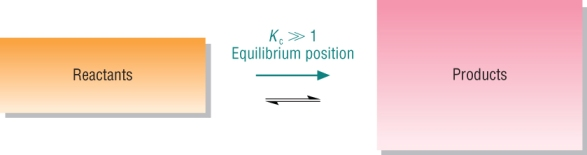Reactants favoured:    Kc < 1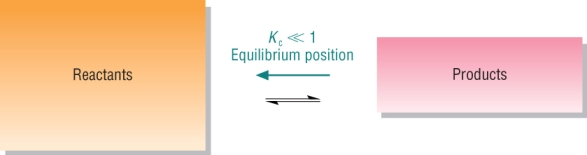How do changes in temperature affect Kc?

• In AS we used Le Chatelier's Principle to predict the how temperature affects the position of equilibrium.
• We can use this to predict what happens to Kc.
• Apply the direction movement to the formula to work out whether Kc increases or decreases:

1)  Endothermic reactions: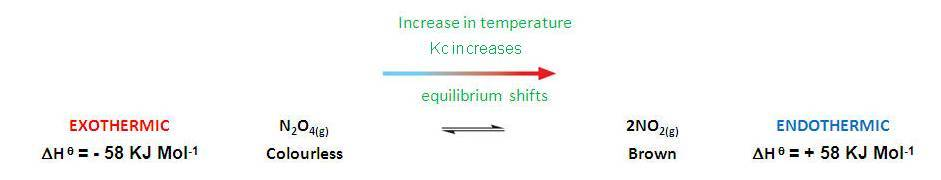• Applying LCP means that an increase in temperature will shift the equilibrium to the Products.
• This means there will be more Products and less Reactants:The top number increases and the bottom number decreases. This makes Kc a larger value.                             Kc increases                             and vice versa

2)  Exothermic reactions: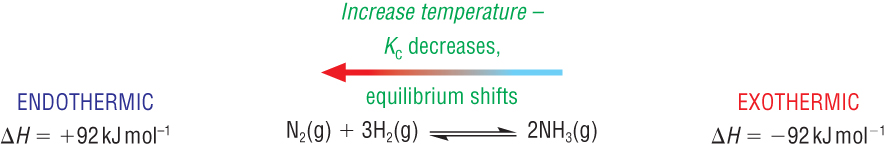• Applying LCP means that an increase in temperature will shift the equilibrium to the Reactants.
• This means there will be more Reactants and less Products:The top number decreases and the bottom number increases. This makes Kc a lsmaller value.                             Kc decreases                             and vice versa

Qu 1  P131

The equilibrium constant, Kc, and the rate constant, k

How does a change in concentration and pressure affect Kc?

• In AS we used Le Chatelier's Principle to predict the how concentration and pressure affects the position of equilibrium.
• The reasons for these changes in the position of the equilibrium lie with Kc.

Kc is unaltered by changes in concentration and pressure

1)  Changes in concentration:

A change in Concentration has no effect on the equilibrium constant.

• Remember if a reactant / product is added the equilibrium shifts to the opposite direction to keep the 'proportions' the same - Kc is unchanged:

 Reaction: N2O4(g) D 2NO2(g)
 [NO2] = 1.60 Mol dm-3 [NO2] = 1.60 Mol dm-3 [N2O4] = 0.20 Mol dm-3 Double [  ] : [N2O4] = 0.40 Mol dm-3 Kc = [NO2]2 Kc = [NO2]2 [N2O4] [N2O4] Kc = (1.60)2 Kc = (3.20)2 0.20 0.40 Kc = 12.8 Mol dm-3 Kc = 6.4 Mol dm-3
• If the concentrations are doubled, the system is no longer at equilibrium, Kc = 12.8

• To bring Kc back from 6.4 to 12.8:

• The system must increase [NO2] and decrease [N2O4]

• Remember if a reactant / product is added the equilibrium shifts to the opposite direction to keep the 'proportions' the same - Kc is unchanged:

2)  Changes in Pressure:

A change in Pressure has no effect on the equilibrium constant.

• If pressure is doubled - the volume is halved -  meaning that the concentrations will have doubled

 Reaction: N2O4(g) D 2NO2(g)
 [NO2] = 1.60 Mol dm-3 Double [  ] : [NO2] = 3.20 Mol dm-3 [N2O4] = 0.20 Mol dm-3 [N2O4] = 0.40 Mol dm-3 Kc = [NO2]2 Kc = [NO2]2 [N2O4] [N2O4] Kc = (1.60)2 Kc = (3.20)2 0.20 0.40 Kc = 12.8 Mol dm-3 Kc = 25.6 Mol dm-3
• If the pressure is doubled, the system is no longer at equilibrium, Kc = 12.8

• To bring Kc back from 25.6 to 12.8:

• The system must increase [NO2] and decrease [N2O4]

• Remember if a reactant / product is added the equilibrium shifts to the opposite direction to keep the 'proportions' the same - Kc is unchanged:

How does the presence of a catalyst affect Kc?

A catalyst has no effect on the equilibrium constant.

• A catalyst speeds up both the forward and reverse reaction.

• Equilibrium is achieved more quickly.

The equilibrium constant Kc, and the rate constant, k

• These 2 constants tell us the most important things in the chemical industry:

 a) Equilibrium How far b) Rates How fast

a)  The equilibrium constant, Kc

• Kc indicates the position of the equilibrium:

 Large Kc Products predominate Small Kc Reactants predominate
• Remember LCP:

 Endothermic Kc increases with an increase in temperature (increases products) Exothermic Kc decreases with an increase in temperature (decreases products)

Kc can be written from the balanced chemical equation

b)  The rate constant, k

• k is a measure of the rate of a reaction:

 Large k Fast rate Small k Slow rate
• Remember:

 k increases with an increase in temperature - Rate increases with an increase in temperature k decreases with a decrease in temperature - Rate decreases with a decrease in temperature

k can only be determined experimentally from the rate equation

The importance of compromise:

• The 2 desirable outcomes are a)  increasing rate and b)  increasing the amount:

a)  Increasing the rate:

 Increasing temperature:  Increases the rate of production of product - desirable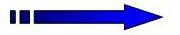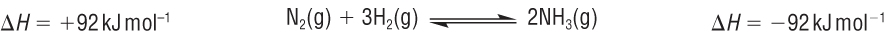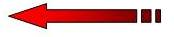Increasing temperature:  Decreases the amount of product made - undesirable

b)  Increasing the amount:

 Decreasing the temperature:  Increases the amount of product made - desirableDecreasing temperature:  Decreases the rate of production of product - undesirable

The compromise is:

 Moderate temperature: k increases moderately - Rate increases by a moderate amount Moderate temperature: Kc decreases by a moderate amount - allowing a moderate yield

Qu 1  P133  /  Qu 4 - 5  P159  /  Qu 3 - 4  P161